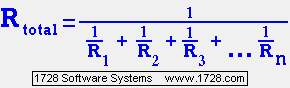Resistors In Parallel Calculator If you are looking for a resistor COLOR CODE calculator, then click here.This calculator can determine the resistance of up to 10 resistors in parallel. Enter resistances into the boxes below and when all values have been input, click on the CALCULATE button and the result will appear in the box below that button. As a test, if you input resistances of 3, 9 and 18 ohms, your answer should be 2 ohms.
 Clicking the RESET button clears all the boxes.

This calculator can solve other math problems.
Calculating resistors in parallel is PRECISELY the same as the calculations required for INDUCTORS in PARALLEL or for CAPACITORS in SERIES.

This calculator can be used for work problems. For example, 'A' can paint a room in 5 hours and 'B' can paint a room in 6 hours. If they both work together how long will the job take? Input the 5 and 6 just as if they were resistors and get your answer.

This calculator can be used for 'fill' problems. For example, one pipe can fill a water tank in 5 hours, while another pipe can fill the same tank in 6 hours. If both pipes are working at the same time........hmmm seems eerily familiar to the other problem doesn't it?

Good luck with your math problems.

Significant Figures >>>
Numbers are displayed in scientific notation with the amount of significant figures you specify. For easier readability, numbers between .001 and 1,000 will not be in scientific notation but will still have the same precision.
You may change the number of significant figures displayed by changing the number in the box above.
Most browsers, will display the answers properly but if you are seeing no answers at all, enter a zero in the box above, which will eliminate all formatting but at least you will see the answers.